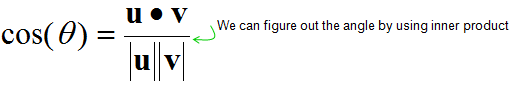Engineering Math - Quick Reference                                 Home : www.sharetechnote.com Matrix - Scalar Triple Product   We have learned very important two operations for vectors, inner product and outer product. Combining these two operation we can produce extremely useful tool. This tool is so widely used that it has it's own name, called 'Scalar Triple Product'.   Let me give you a situation where we can use the concept of Scalar Triple Product. We have a multiple planes connected to each other with different tilt and light (sun) is shining over them as shown below.   Now let's think about which plane (plate) would have the strongest light. Any idea ? Intuitively you would guess that the plate getting the light closer to right angle (perpendicular to the plane/plate).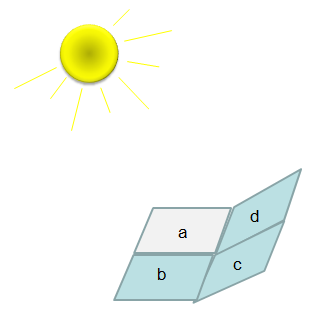How can we know how closer to the right angle a plate is getting the light ? The idea is like this. i) Draw a vector which is perpendicular to the plane/plate(Let's label this vector as s). ii) Draw a vector which connector a corner of the plate to the center of the light(Let's lavel this vector as l ) iii) Calculate the angle between the two vectors (s and l). If this angle closer to 0, it means the it is getting the light closer to the right angle.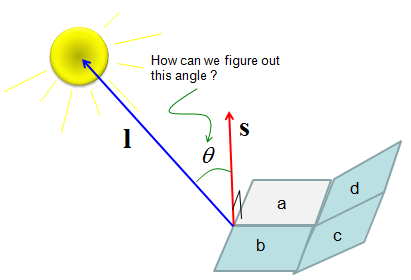Now let translate this procedure into mathematical forms. First, we have to get a vector which is perpendicular (normal) to a plane (plate). Any idea on this ? This is where you can use the concept of 'Cross Product'. If you draw two vectors starting a corner of the plate running along the sides as marked in green arrow shown below. The cross product of the two vectors would give you a vector which is perpendicular to the plate. Now the next step would be to figure out the angle between the angle between vector s and l. This is what Inner Product is used for.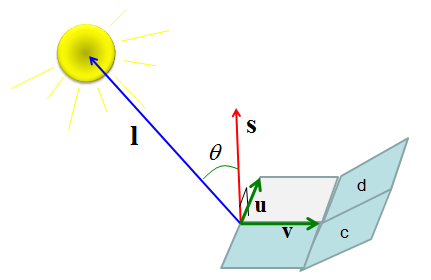Following is the mathematical description for the procedures explained above.   First, get the vector s which is perpendicular to a plate by 'Cross Product' as shown below.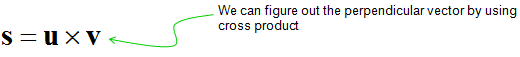Second, by a little bit of rearrangement of Inner Product equation, you can get the angles between the vector s and vector l.